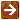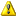﻿ Cash Available and Cash Needed Calculations

#### Cash Available and Cash Needed CalculationsUnless otherwise indicated, all calculations adhere to the standard rounding rules. For information on those rules, see Standard Rounding Rules.

If excess income or principal cash is available, the process directs the system to calculate how many units of a security to purchase. Purchases are made at the current unit value for each pool. The system obtains the current unit value from the security record for each pooled fund in the model and calculates the number of units to purchase as follows.

1.     Using the percentages specified in the model, calculates the amount of cash available for purchase of each security (pooled fund).

2.     Using the calculated amount available for purchase for each pooled fund, divides the amount by the pooled fund unit value to obtain the number of units of each pool to purchase.

3.     If the purchase of fractional shares is not allowed in the model, rounds the result down to the nearest whole number to ensure that the cash equivalents are not overdrawn .The rule noted above is a non-standard rounding rule.

4.     Calculates the actual purchase amount for each pooled fund by taking the number of purchase units times the unit value.

5.     Rounds the result to two decimals as the actual purchase amount.

If available income or principal cash funds are less than zero, the system calculates the number of units to sell. Sales are made at the current unit value for each pool. It obtains the current unit value from the security record for each pooled fund in the model and calculates the number of units to sell as follows.

1.     Using the percentages specified in the model, calculates the amount of cash needed from the sale of each security (pooled fund).

2.     Using the calculated amount needed for sale of each pooled fund, divides the amount by the pooled fund unit value to obtain the number of units of each pool to sell.

3.     If the sale of fractional shares is not allowed in the model, rounds the result up to the nearest whole number to ensure that adequate cash is withdrawn.The rule noted above is a non-standard rounding rule.

4.     Calculates the actual amount to be sold from each pooled fund by taking the number of units sold times the unit value.

5.     Rounds the result to two decimals as the actual sold amount.

More: